Also found in: Dictionary, Thesaurus, Wikipedia.

[′kwä·drik ′sər·fəs]
(mathematics)
A surface whose equation is a second-degree algebraic equation.

a surface such that the rectangular Cartesian coordinates of its points satisfy the second-degree algebraic equation

(*) a1 1x2 + a2 2y2 + a3 3z2 + 2a1 2xy + 2a2 3xy + 2a1 3xz + 2a1 4x + 2a2 4y + 2a3 4z + a4 4 = 0

If equation (*) does not define a real geometric locus, in order to preserve generality we say that it defines an imaginary quadric surface. Depending on the values of the coefficients of equation (*), it can be transformed by means of translation and rotation of axes into one of the 17 canonical forms listed below. A specific class of quadric surfaces corresponds to each of these forms. Five principal types of such surfaces are distinguished:

(1) Ellipsoids: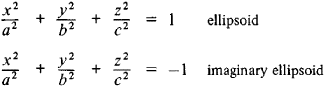(2) Hyperboloids: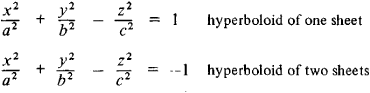(3) Paraboloids (p > 0, q> 0):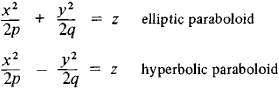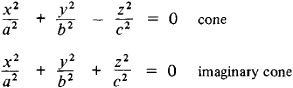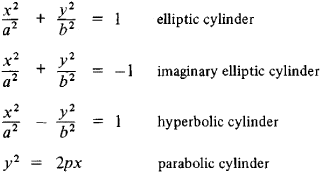The invariants of the general equation of a quadric surface— that is, expressions that are formed from the coefficients of equation (*) and that do not change in value upon translation or rotation of the axes—are of great importance in the investigation of the general equation of a quadric surface. For example, if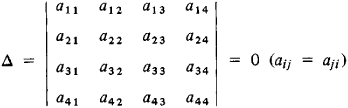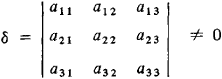then the surface has a unique center of symmetry called the center of the quadric surface and is said to be central. If δ = 0, then the surface either does not have a center or has infinitely many centers.

Affine and projective classifications have been established for quadric surfaces. Two quadric surfaces are considered to belong to the same affine class if they can be transformed into each other by an affine transformation; projective classes of quadric surfaces are defined in a similar manner. To each affine class there corresponds one of the 17 canonical forms of the equation of a quadric surface. Projective transformations permit the relation between different affine classes of quadric surfaces to be established because for these transformations the special role of infinitely distant elements of space vanishes. For example, hyperboloids of two sheets and ellipsoids, which are different from the affine point of view, belong to the same projective class of quadric surfaces.

### REFERENCES

Aleksandrov, P. S. Lektsii po analiticheskoi geometrii…. Moscow, 1968.
Il’in, V. A., and E. G. Pozniak. Analiticheskaia geometriia, 2nd ed. Moscow, 1971.
Efimov, N. V. Kvadratichnye formy i matritsy, 5th ed. Moscow, 1972.

A. B. IVANOV

References in periodicals archive ?
The quadric surface we discuss in this paper will be an ellipsoid, an elliptic paraboloid, a hyperboloid of two sheets or a cone; however, we will leave it to reader to explore the case when quadric surface is a cone.
The equation of the quadric surface, we use in the form of (1), is equivalent to (7), (9) and (10).
Each of the roots of the quadratic equation (12) corresponds to one of the axes of the quadric surface, we may use either solution for further investigation.
Let us name the solid bounded by the quadric surface and the plane [S.
Our goal now is to find the volume of the solid of the intersection between the quadric surface and the plane by using spatial spherical coordinates.
We may assume that the calculation accuracy increases if point is located near the center of the intersection curve of the quadric surface and the plane.
We first discuss a general strategy of how we may use the Divergence theorem to find the volume of the solid bounded by a quadric surface and a plane.
Our focus now is to apply the Stokes' theorem to find the volume of the solid bounded by the quadric surface and the plane.
and the right side of (66) contains the surface integral over the entire surface of the quadric surface.

Site: Follow: Share:
Open / Close